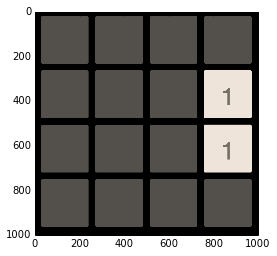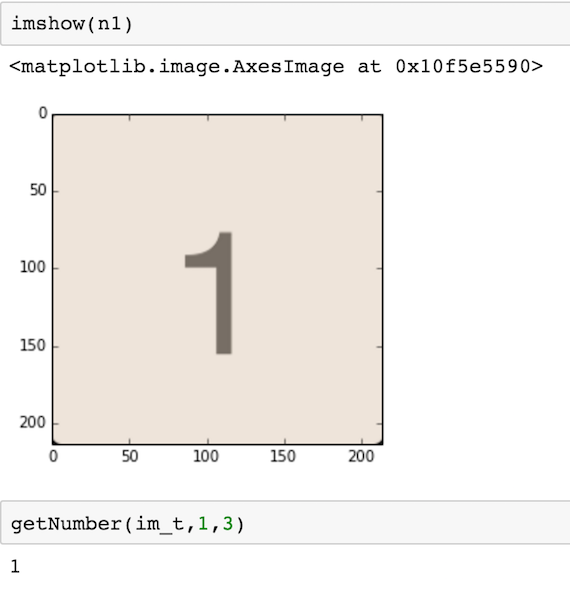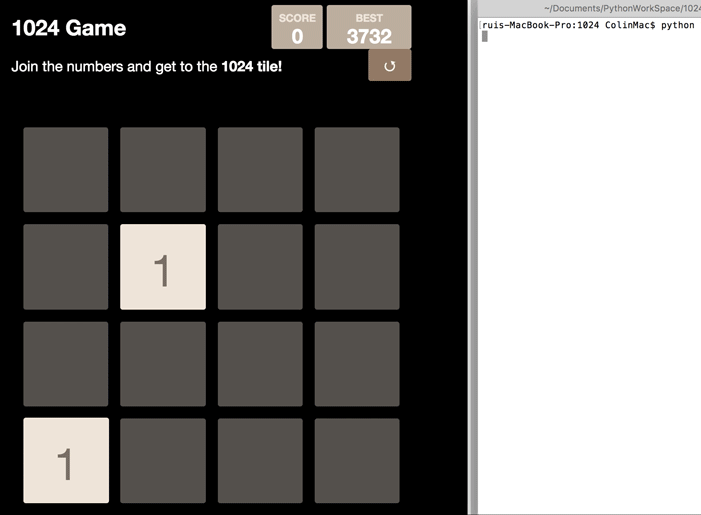小游戏1024自动机与AI第一步 图形抓取

import ImageGrab
# initial graphic settings
BOX = (55,545,1055,1545)
WIDTH = 214
INTERVAL = 28
# position checking
im_t = np.asarray(ImageGrab.grab(BOX))
imshow(im_t)
# get origin_x, origin_y
origin_x, origin_y = getOrigin(im_t)
print 'origin =', origin_x, origin_y
origin = 27 23第二步 数字识别第三步 模拟游戏

# initial board
board = np.zeros((4,4), dtype=np.int)
for x in range(4):
for y in range(4):
board[x][y] = getNumber(im_t,x,y) or 0
print board
[[0 0 0 0]
[0 0 0 1]
[0 0 0 1]
[0 0 0 0]]

def moveRight(board):
board = np.fliplr(board)
moveLeft(board)
board = np.fliplr(board)
def moveUp(board):
board = np.rot90 (board)
moveLeft(board)
board = np.rot90 (board,3)
def moveDown(board):
board = np.rot90 (board,3)
moveLeft(board)
board = np.rot90 (board)

Gamve Over的判定：

第四步 AI算法

if np.array_equal(lastBoard,board):
return -1000
rst = 0
rst += 5 * len(filter(lambda x: x ==0, board.flatten()))
rst += 10 * math.log(maxValue(board),2)
temp = np.log2(board+1)
for x in range(4):
for y in range(4):
if y+1 < 4:
rst -= abs(temp[x][y] - temp[x][y+1])
if x+1 < 4:
rst -= abs(temp[x][y] - temp[x+1][y])
rst += temp[x][y]*temp[x][y]
return rst

第五步 与游戏交互

AI帮忙算出了每一步走哪个方向好，我们要把这个决定用指令发送给游戏。

Windows下用ctypes.windll.user32.SendInput很简单，mac上又麻烦了一些。首先搜到的居然是让我用PyObjC调用ObjC来生成按键事件。。。太绕了，打死不从，我们按键的频率又不高。后来找到了用Apple Script的方法，简单直观了不少。

def pressKey(key):
left = 'osascript -e \'tell application "System Events" to key code 123\''
right = 'osascript -e \'tell application "System Events" to key code 124\''
up = 'osascript -e \'tell application "System Events" to key code 126\''
down = 'osascript -e \'tell application "System Events" to key code 125\''
if key == 0:
os.system(left)
if key == 1:
os.system(right)
if key == 2:
os.system(up)
if key == 3:
os.system(down)

第六步 测试github地址